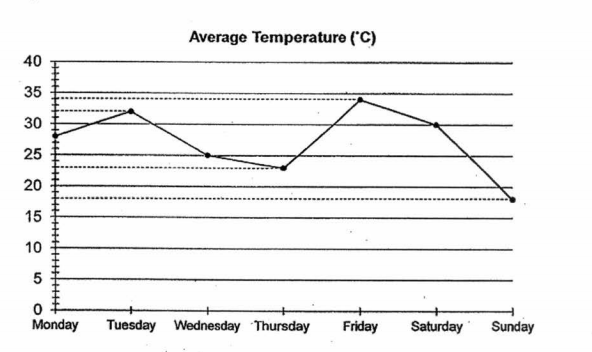# Primary 6 SA2 Practice #6

Time elapsed:
Q1. Which one of the following numbers is nearest to 8?     [1 mark]
Q2. Express 8005 m in kilometres and metres.     [1 mark]
Q3. Which of the following is the same as 6.05 km?     [1 mark]
Q4. Which of the following fractions is larger than 15?     [1 mark]
Q5. Express 0.507 as a percentage.     [1 mark]
Q6. Daisy bought a speaker and a laptop. She spent \$2000 altogether. The speaker is 4% of the total cost. What is the cost of the laptop?     [2 marks]
Q7. In the scale below, what is the value of A?     [1 mark]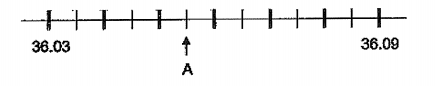Q8. William spent \$50 of his allowance and saved the rest. When he increased his spending by 10%, his saving decreased by 20%. How much was his allowance?     [2 marks]
Q9. The figure below is made up of two identical quarter circles of radius 3 cm. What is the perimeter of the figure?     [2 marks]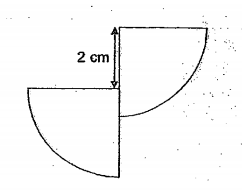Q10. Express 1 036 millilitres in litres.     [1 mark]
Q11. Which digit in 69.87 is in the tenths place?     [1 mark]
Q12. The graph shows the number of chairs sold by a shop from May to August. In which two months were a total of 76 chairs sold?     [1 mark]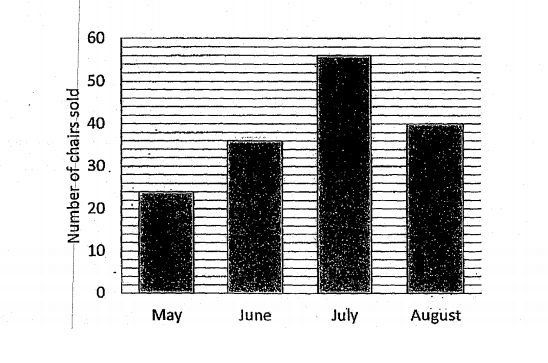Q13. Express 700 g in kilogram.     [1 mark]
Q14. Which of the following numbers is the smallest?     [1 mark]
Q15. Which of the following is the same as 9.04 ℓ?     [1 mark]
Q16. The figure below is made up of a square, a quadrant and a circle. The length of the square is 14 cm. Find the perimeter of the shaded portion of the figure. (Take π = 22/7)     [2 marks]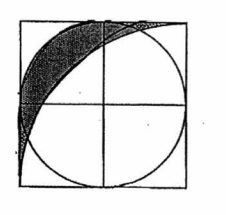Q17. The bar graph below shows the time taken by 4 boys to complete a race. Which boy finished third in the race?     [1 mark]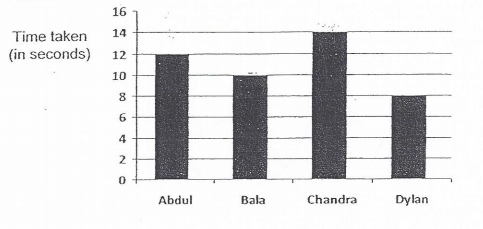Q18. In the figures below, O is the centre of the circle and the circumference of the circle is the same as the perimeter of the square. What is the area of the square? (Take π = 22/7)     [2 marks]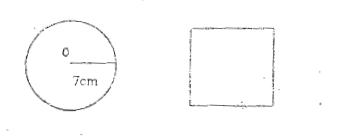Q19. Isabelle bought some sugar. She used 13 of the sugar on Monday and 25 of it on Tuesday. The amount of sugar she had left was 132 g. How much sugar did Isabelle buy?     [2 marks]
Q20. The graph below shows the average temperature from Monday to Sunday. From which day to which day was there the greatest drop in temperature?     [1 mark]